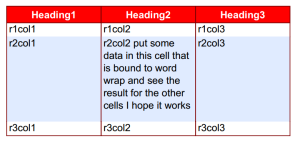Select Page

# TCPDF Tables with Word Wrapping Cells#### October 3, 2014

This code is used to create a table using TCPDF the example I got it from used Cell which didn’t word wrap and then when switching to writeCell the borders were messed up so I had to change the code from another example to properly write the cells and their borders.

This is the result:I’m using CakePHP

```App::import('Vendor','xtcpdf');

\$pdf = new XTCPDF(PDF_PAGE_ORIENTATION, PDF_UNIT, PDF_PAGE_FORMAT, true, 'UTF-8', false);
\$pdf->ColoredTable(
array(40,40,40),
array(
array('r1col1', 'r1col2', 'r1col3'),
array('r2col1', 'r2col2 put some data in this cell that is bound to word wrap and see the result for the other cells I hope it works', 'r2col3'),
array('r3col1', 'r3col2', 'r3col3')
)
);

```

The XTCPDF class is in Vendor/xtcpdf.php and TCPDF is in Vendor/tcpdf

```App::import('Vendor','tcpdf/tcpdf');
class XTPDF extends TCPDF
{

/* function ColoredTable
* @param \$w array of column widths array(40, 30,70);
* @param array of data array( array( r1col1, r1col2, r1col3), array(r2col1, r2col2, r3col3))
*/

// Colors, line width and bold font
\$this->SetFillColor(255, 0, 0);
\$this->SetTextColor(255);
\$this->SetDrawColor(128, 0, 0);
\$this->SetLineWidth(0.3);
\$this->SetFont('', 'B');

for(\$i = 0; \$i < \$num_headers; ++\$i) {
\$this->Cell(\$w[\$i], 7, \$header[\$i], 1, 0, 'C', 1);
}
\$this->Ln();
// Color and font restoration
\$this->SetFillColor(224, 235, 255);
\$this->SetTextColor(0);
\$this->SetFont('');
// Data

\$fill = 0;

foreach(\$data as \$row) {

\$cellcount = array();
//write text first
\$startX = \$this->GetX();
\$startY = \$this->GetY();
//draw cells and record maximum cellcount
//cell height is 6 and width is 80

foreach (\$row as \$key => \$column):
\$cellcount[] = \$this->MultiCell(\$w[\$key],6,\$column,0,'L',\$fill,0);
endforeach;

\$this->SetXY(\$startX,\$startY);

//now do borders and fill
//cell height is 6 times the max number of cells

\$maxnocells = max(\$cellcount);

foreach (\$row as \$key => \$column):
\$this->MultiCell(\$w[\$key],\$maxnocells * 6,'','LR','L',\$fill,0);
endforeach;

\$this->Ln();
// fill equals not fill (flip/flop)
\$fill=!\$fill;

}

// draw bottom row border
\$this->Cell(array_sum(\$w), 0, '', 'T');
}
```

1.Hello James, this help me a lot, thanks. but there’s a issue in the code, if you have some data that occupies more than a single page, the loop writes one single line of the next page, overwriting himself until the end of the loop.

•this code can fix this:
``` \$i += \$maxnocells; if (\$i > 23) { \$this->AddPage('L', 'A4'); \$i = 0; } ```
edit the condition of the \$i in order of your paper type (my example use Landscape A4 )

•Thank you so much!

•Only 5 years after your post. Thanks for this. Didn’t hit this bug because I never made a big table with this code but thanks for posting the fix. Will help me the next time I use it!

2.Dear James,

Maybe you can help me out with tcpdf in landscape orientation and a QR code. I am having trouble with the layout when i turn the tcpdf in landscape.

For example:

\$pdf->SetAutoPageBreak(false, 0);

// new style
\$style = array(
‘border’ => false,
‘fgcolor’ => array(0,0,0),
‘bgcolor’ => false
);

\$pdf->write2DBarcode(‘http://www.google.com/’, ‘QRCODE,H’, 0, 1, 100, 100, \$style, ‘N’);

doesn’t work and just

Will display a bigger QRcode

•Check out the docs https://tcpdf.org/docs/srcdoc/TCPDF/class-TCPDF/

write2DBarcode( \$code, \$type, \$x = ”, \$y = ”, \$w = ”, \$h = ”, \$style = array(), \$align = ”, \$distort = false )

edit the x and y and the location of the barcode on the page will change and edit w and h and the size of the barcode will change to suit

\$style = [
‘border’ => false,